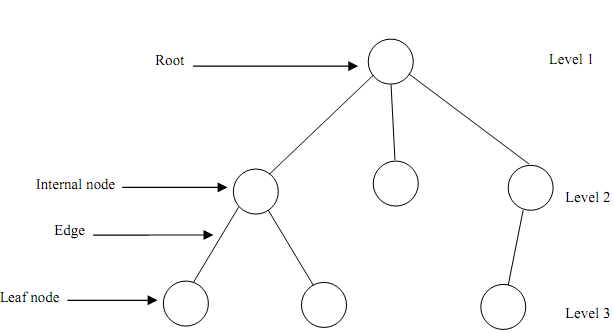## Rooted tree, Data Structure & Algorithms

Assignment Help:

It does not have any cycles (circuits, or closed paths), which would imply the existence of more than one path among two nodes. It is the most general kind of tree, and might be converted in the more familiar form though designating a node as the root. We can represent a tree like a construction containing nodes, and edges that represent a relationship among two nodes. In Figure, we will assume most common tree called rooted tree. A rooted tress has a single root node that has no parents.Figure: A rooted tree

In more formal way, we can define tree T like a finite set of one or more nodes such that there is one designated node r called as the root of T, and the remaining nodes into (T - { r } ) are partitioned in n > 0 disjoint subsets T1, T2, ..., Tk  each of is a tree, and whose roots r1 , r2 , ..., rk , respectively, are children of r. The general tree is a generic tree which has one root node, and each node in the tree can have limitless number of child nodes. One popular employ of this kind of tree is a Family Tree.

A tree is an example of a more general category called graph.

• A tree contains nodes connected by edges.
• A root is node without parent.
• Leaves are nodes having no children.
• The root is at level 1. The child nodes of root are at level 2. The child nodes of nodes at level 2 are at level 3 and so forth.
• The depth (height) of any Binary tree is equivalent to the number of levels in it.
• Branching factor describe the maximum number of children to any node. Thus, a branching factor of 2 means a binary tree.
• Breadth described the number of nodes at a level.
• In a tree the depth of a node M is the length of the path from the root of the tree to M.
• In a Binary tree a node has at most 2 children. The given are the properties of a Tree.

Full Tree: A tree having all the leaves at the similar level, and all the non-leaves having the similar degree

• Level h of a full tree contains dh-1 nodes.
• The first h levels of full tree have 1 + d + d2 + d3 + d4 + ....... + dh-1 = (dh -1)/(d - 1) nodes where d refer to the degree of nodes.
• The number of edges = the number of nodes - 1 (Why? Because, an edge represents the relationship among a child & a parent, and every node has a parent except the root.
• A tree of height h & degree d has at most d h - 1 element.

#### Related Discussions:- Rooted tree

Compare zero-address, one-address, two-address, and three-address machines by writing programs to compute: Y = (A – B X C) / (D + E X F) for each of the four machines. The inst

#### Two - way merge sort, Merge sort is also one of the 'divide & conquer' clas...

Merge sort is also one of the 'divide & conquer' classes of algorithms. The fundamental idea in it is to split the list in a number of sublists, sort each of these sublists & merge

#### Infix expression has balanced parenthesis or not, Q. By making use of stack...

Q. By making use of stacks, write an algorithm to determine whether the infix expression has balanced parenthesis or not.

#### B-tree of degree 3, Q. Explain the result of inserting the keys given. ...

Q. Explain the result of inserting the keys given. F, S, Q, K, C, L, H, T, V, W, M, R, N, P, A, B, X, Y, D, Z, E  in an order to an empty B-tree of degree-3.

#### Breadth-first search, Breadth-first search starts at a given vertex h, whic...

Breadth-first search starts at a given vertex h, which is at level 0. In the first stage, we go to all the vertices that are at the distance of one edge away. When we go there, we

#### Examination, Write an algorithm for binary search. What are its limitations...

Write an algorithm for binary search. What are its limitations? .

#### Algorithm that inputs the codes for all items in stock, A shop sells books,...

A shop sells books, magazines and maps. Every item is identified by a unique 4 - digit code. All books have a code which starts with 1, all maps have a code starting with 2 and all

#### Queues, what is the difference between data type and abstract data type

what is the difference between data type and abstract data type

#### Dqueue, algorithm of output restricted queue.

algorithm of output restricted queue.

#### Relation of time and space complexities of an algorithm, What is complexity...

What is complexity of an algorithm? What is the basic relation between the time and space complexities of an algorithm? Justify your answer by giving an example.